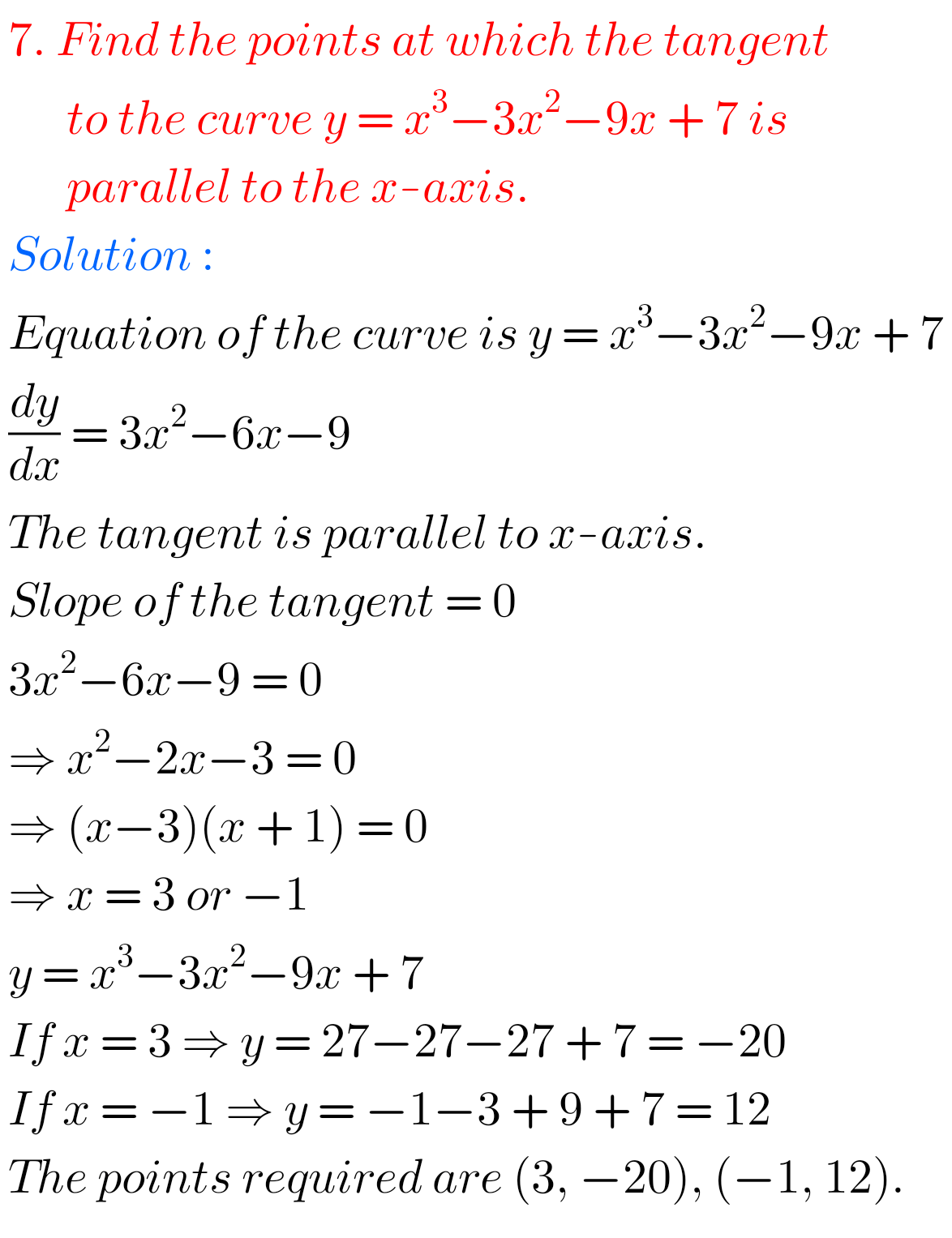## Applications of Derivatives Exercise 10(b) solutions Inter Maths 1B

Intermediate Maths 1B solutions for Applications of Derivatives exercise 10(b) solutions Applications of Derivatives exercise 10(b) Maths 1B textbook Inter solutions are given. Study the textbook lesson Applications of Derivatives very well. Observe the example problems and solutions given in the textbook. Observe the given below solutions and try them in your own method. You …